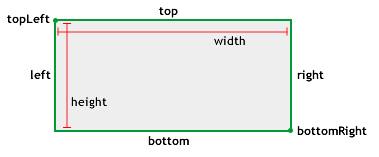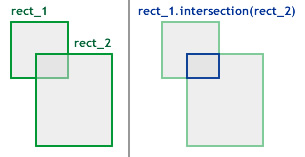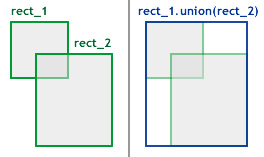A Rectangle object is an area defined by its position, as indicated by its top-left corner point(x, y) and by its width and its height.

The `x`, `y`, `width`, and `height` properties of the Rectangle class are independent of each other; changing the value of one property has no effect on the others. However, the `right` and `bottom` properties are integrally related to those four properties. For example, if you change the value of the `right` property, the value of the `width` property changes; if you change the `bottom` property, the value of the `height` property changes.

The following methods and properties use Rectangle objects:

• The `applyFilter()`, `colorTransform()`, `copyChannel()`, `copyPixels()`, `draw()`, `fillRect()`, `generateFilterRect()`, `getColorBoundsRect()`, `getPixels()`, `merge()`, `paletteMap()`, `pixelDisolve()`, `setPixels()`, and `threshold()` methods, and the `rect` property of the BitmapData class
• The `getBounds()` and `getRect()` methods, and the `scrollRect` and `scale9Grid` properties of the DisplayObject class
• The `getCharBoundaries()` method of the TextField class
• The `pixelBounds` property of the Transform class
• The `bounds` parameter for the `startDrag()` method of the Sprite class
• The `printArea` parameter of the `addPage()` method of the PrintJob class

You can use the `new Rectangle()` constructor to create a Rectangle object.

Note: The Rectangle class does not define a rectangular Shape display object. To draw a rectangular Shape object onscreen, use the `drawRect()` method of the Graphics class.

### `new (x:Float = 0, y:Float = 0, width:Float = 0, height:Float = 0)`

Creates a new Rectangle object with the top-left corner specified by the `x` and `y` parameters and with the specified `width` and `height` parameters. If you call this function without parameters, a rectangle with `x`, `y`, `width`, and `height` properties set to 0 is created.

Parameters:

`x` The x coordinate of the top-left corner of the rectangle. The y coordinate of the top-left corner of the rectangle. The width of the rectangle, in pixels. The height of the rectangle, in pixels.

### `bottom:Float`

The sum of the `y` and `height` properties.### `bottomRight:Point`

The location of the Rectangle object's bottom-right corner, determined by the values of the `right` and `bottom` properties.### `height:Float`

The height of the rectangle, in pixels. Changing the `height` value of a Rectangle object has no effect on the `x`, `y`, and `width` properties.### `left:Float`

The x coordinate of the top-left corner of the rectangle. Changing the `left` property of a Rectangle object has no effect on the `y` and `height` properties. However it does affect the `width` property, whereas changing the `x` value does not affect the `width` property.

The value of the `left` property is equal to the value of the `x` property.### `right:Float`

The sum of the `x` and `width` properties.### `size:Point`

The size of the Rectangle object, expressed as a Point object with the values of the `width` and `height` properties.

### `top:Float`

The y coordinate of the top-left corner of the rectangle. Changing the `top` property of a Rectangle object has no effect on the `x` and `width` properties. However it does affect the `height` property, whereas changing the `y` value does not affect the `height` property. The value of the `top` property is equal to the value of the `y` property.### `topLeft:Point`

The location of the Rectangle object's top-left corner, determined by the x and y coordinates of the point.### `width:Float`

The width of the rectangle, in pixels. Changing the `width` value of a Rectangle object has no effect on the `x`, `y`, and `height` properties.### `x:Float`

The x coordinate of the top-left corner of the rectangle. Changing the value of the `x` property of a Rectangle object has no effect on the `y`, `width`, and `height` properties.

The value of the `x` property is equal to the value of the `left` property.

### `y:Float`

The y coordinate of the top-left corner of the rectangle. Changing the value of the `y` property of a Rectangle object has no effect on the `x`, `width`, and `height` properties.

The value of the `y` property is equal to the value of the `top` property.

### `clone ():Rectangle`

Returns a new Rectangle object with the same values for the `x`, `y`, `width`, and `height` properties as the original Rectangle object.

Returns:

A new Rectangle object with the same values for the `x`, `y`, `width`, and `height` properties as the original Rectangle object.

### `contains (x:Float, y:Float):Bool`

Determines whether the specified point is contained within the rectangular region defined by this Rectangle object.

Parameters:

`x` The x coordinate(horizontal position) of the point. The y coordinate(vertical position) of the point.

Returns:

A value of `true` if the Rectangle object contains the specified point; otherwise `false`.

### `containsPoint (point:Point):Bool`

Determines whether the specified point is contained within the rectangular region defined by this Rectangle object. This method is similar to the `Rectangle.contains()` method, except that it takes a Point object as a parameter.

Parameters:

`point` The point, as represented by its x and y coordinates.

Returns:

A value of `true` if the Rectangle object contains the specified point; otherwise `false`.

### `containsRect (rect:Rectangle):Bool`

Determines whether the Rectangle object specified by the `rect` parameter is contained within this Rectangle object. A Rectangle object is said to contain another if the second Rectangle object falls entirely within the boundaries of the first.

Parameters:

`rect` The Rectangle object being checked.

Returns:

A value of `true` if the Rectangle object that you specify is contained by this Rectangle object; otherwise `false`.

### `copyFrom (sourceRect:Rectangle):Void`

Copies all of rectangle data from the source Rectangle object into the calling Rectangle object.

Parameters:

`sourceRect` The Rectangle object from which to copy the data.

### `equals (toCompare:Rectangle):Bool`

Determines whether the object specified in the `toCompare` parameter is equal to this Rectangle object. This method compares the `x`, `y`, `width`, and `height` properties of an object against the same properties of this Rectangle object.

Parameters:

`toCompare` The rectangle to compare to this Rectangle object.

Returns:

A value of `true` if the object has exactly the same values for the `x`, `y`, `width`, and `height` properties as this Rectangle object; otherwise `false`.

### `inflate (dx:Float, dy:Float):Void`

Increases the size of the Rectangle object by the specified amounts, in pixels. The center point of the Rectangle object stays the same, and its size increases to the left and right by the `dx` value, and to the top and the bottom by the `dy` value.

Parameters:

`dx` The value to be added to the left and the right of the Rectangle object. The following equation is used to calculate the new width and position of the rectangle: ``````x -= dx; width += 2 * dx;`````` The value to be added to the top and the bottom of the Rectangle. The following equation is used to calculate the new height and position of the rectangle: ``````y -= dy; height += 2 * dy;``````

### `inflatePoint (point:Point):Void`

Increases the size of the Rectangle object. This method is similar to the `Rectangle.inflate()` method except it takes a Point object as a parameter. The following two code examples give the same result:

``````var rect1 = new Rectangle(0,0,2,5);
rect1.inflate(2,2);``````
``````var rect1 = new Rectangle(0,0,2,5);
var pt1 = new Point(2,2);
rect1.inflatePoint(pt1);``````

Parameters:

`point` The `x` property of this Point object is used to increase the horizontal dimension of the Rectangle object. The `y` property is used to increase the vertical dimension of the Rectangle object.

### `intersection (toIntersect:Rectangle):Rectangle`

If the Rectangle object specified in the `toIntersect` parameter intersects with this Rectangle object, returns the area of intersection as a Rectangle object. If the rectangles do not intersect, this method returns an empty Rectangle object with its properties set to 0.Parameters:

`toIntersect` The Rectangle object to compare against to see if it intersects with this Rectangle object.

Returns:

A Rectangle object that equals the area of intersection. If the rectangles do not intersect, this method returns an empty Rectangle object; that is, a rectangle with its `x`, `y`, `width`, and `height` properties set to 0.

### `intersects (toIntersect:Rectangle):Bool`

Determines whether the object specified in the `toIntersect` parameter intersects with this Rectangle object. This method checks the `x`, `y`, `width`, and `height` properties of the specified Rectangle object to see if it intersects with this Rectangle object.

Parameters:

`toIntersect` The Rectangle object to compare against this Rectangle object.

Returns:

A value of `true` if the specified object intersects with this Rectangle object; otherwise `false`.

### `isEmpty ():Bool`

Determines whether or not this Rectangle object is empty.

Returns:

A value of `true` if the Rectangle object's width or height is less than or equal to 0; otherwise `false`.

### `offset (dx:Float, dy:Float):Void`

Adjusts the location of the Rectangle object, as determined by its top-left corner, by the specified amounts.

Parameters:

`dx` Moves the x value of the Rectangle object by this amount. Moves the y value of the Rectangle object by this amount.

### `offsetPoint (point:Point):Void`

Adjusts the location of the Rectangle object using a Point object as a parameter. This method is similar to the `Rectangle.offset()` method, except that it takes a Point object as a parameter.

Parameters:

`point` A Point object to use to offset this Rectangle object.

### `setEmpty ():Void`

Sets all of the Rectangle object's properties to 0. A Rectangle object is empty if its width or height is less than or equal to 0.

This method sets the values of the `x`, `y`, `width`, and `height` properties to 0.

### `setTo (xa:Float, ya:Float, widtha:Float, heighta:Float):Void`

Sets the members of Rectangle to the specified values

Parameters:

`xa` the values to set the rectangle to. heighta

### `union (toUnion:Rectangle):Rectangle`

Adds two rectangles together to create a new Rectangle object, by filling in the horizontal and vertical space between the two rectangles.Note: The `union()` method ignores rectangles with `0` as the height or width value, such as: `var rect2:Rectangle = new Rectangle(300,300,50,0);`

Parameters:

`toUnion` A Rectangle object to add to this Rectangle object.

Returns:

A new Rectangle object that is the union of the two rectangles.